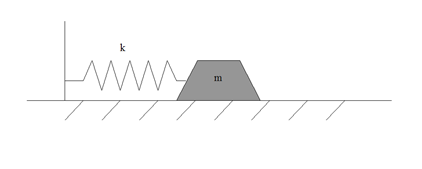## Monday, April 02, 2012

### Math Bait: the Spring-Mass System

To my never ending surprise, one the largest groups of readers Google directs to my humble blog are not policy neophytes seeking enlightenment but rather desperate statistics students trying to find the variance of a Poisson distribution.

In a naked ploy to draw more math geek traffic, let’s consider a classic problem from ordinary second-order linear differential equations with constant coefficients:  the spring-mass system shown below:We have an object of mass m attached to one end of a coil with spring constant k.  The coil exerts force on the mass in direct proportion to its displacement, so F=-kx.  (The spring is both compressible and extensible.)  Let’s impose the initial condition that the mass is displaced distance x0  and released at time t = 0.  Further, let’s add to the system an external sinusoidal force of amplitude A and radian frequency ω.  Applying Newton’s third law of motion, ΣF = mx’’ (x’’ being the second derivative of x with respect to t; in other words, the acceleration), we obtain the differential equation:

Note that x(0) is the position at t = 0 and x’(0) is the velocity at t = 0.

Before beginning the solution, let’s examine the problem intuitively.  We should expect that the mass will move faster with increasing k and slower with increasing m.  Further, we note that, for a positive value of x0, the spring constant k will be pulling the mass to the left at t=0 while the forcing function will be pushing the mass to the right, since the cosine of zero is positive one.

The first step in solving a differential equation is to find its “natural response”; in other words, the solution without the forcing function.  This solution is called the homogeneous solution xh.  We rewrite the equation thus:

Solutions to second-order DEs with constant coefficients take the form Cest; substituting this solution into the equation and dividing through by est yields the characteristic equation in terms of s:

As expected, we have two values of s, thus the homogeneous solution has the form:

We will find the constants C’1 and C’2 by applying the initial conditions, but first we must find the particular solution (also known as the “forced response”).  Because the forcing function is sinusoidal, we anticipate that the particular solution will take the form:

We will now use the method of undetermined coefficients to find the coefficients of our particular solution.  Calculating the second derivative:

We can then insert xp into the equation:

and solve for the coefficients B and C:

yielding the final particular solution

The general solution is the sum of the homogeneous and particular solutions.  Using Euler’s Relation, we rewrite our homogeneous solution in terms of sines and cosines instead of complex exponentials:

We now can apply the initial conditions to find the values of our remaining constants.

Inserting the constants into the equation yields our final solution:

Forcing at the Resonant Frequency

But wait a second!  What if the frequency of the forcing function is the same as the resonant (i.e. natural) frequency?  That would make k – mω2 = 0, and dividing by zero is a big mathematical no-no.  What to do?

Let’s re-examine our particular solution.  If ω=√(k/m), then we must treat the particular solution as we would a double root, by multiplying it by time t.

Finding the second derivative

and inserting into our equation

yields after substituting ω=√(k/m), and simplifying:

We can then solve for our coefficients to find the particular solution:

The general solution is thus the sum of xh and xp.

Finally, we apply our initial conditions to find the remaining coefficients:

And write the final solution to our spring-mass system when the frequency of the forcing function is the resonant frequency:

Many thanks to the fine folks at CodeCogs for their latex-to-gif converter.Anonymous said...

obviously, you are assuming zero friction.

Dr. Φ said...

Patience, prodigy. In time, all will be revealed.Anonymous said...

I wish I could understand this stuff.